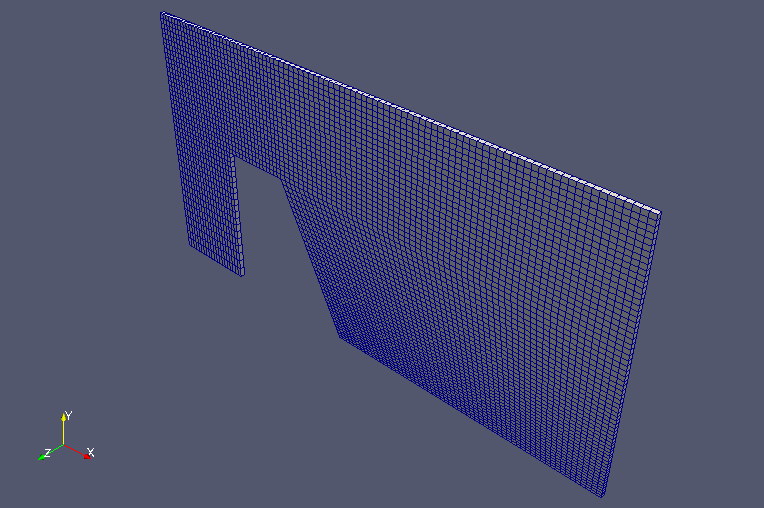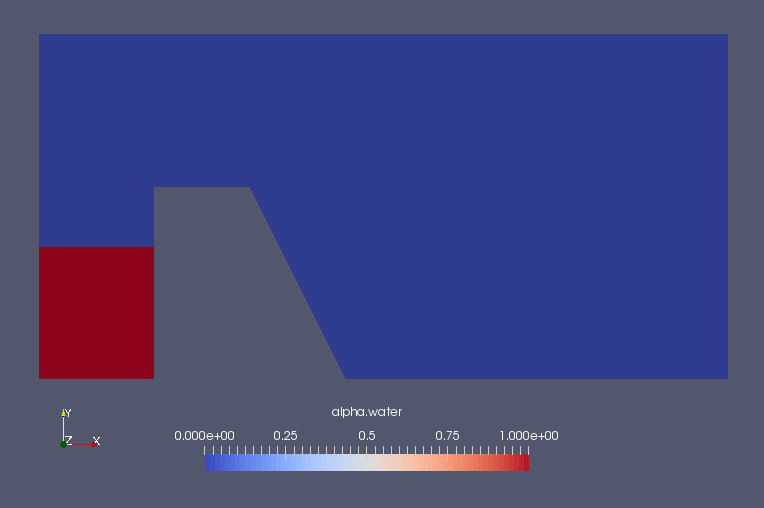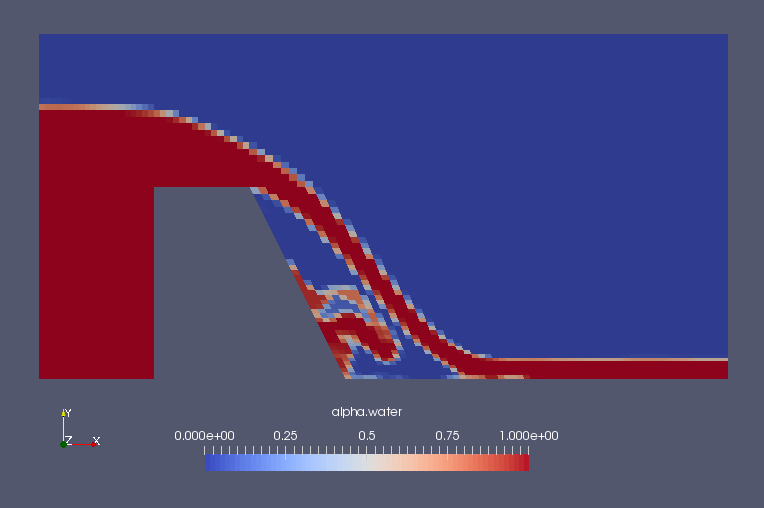﻿ Overflow weir - XSim

# Overflow weir

OpenFOAM 4.x

## Case directory

\$FOAM_TUTORIALS/multiphase/interFoam/ras/weirOverflow

## SummaryModel geometry

We calculate the water flowing over the weir for 60 seconds. Water flows in from the region "inlet" and out from the region "outlet". The weir and the lower surface of the domain (region "lowerWall") are assumed to be under no-slip conditions, and the upper surface of the domain (region "atmosphere") is assumed to be open. The calculation is performed as a two-dimensional problem with a single mesh in the Z direction.

The gravity acceleration is defined in the file "constant/g" as follows.

```dimensions      [0 1 -2 0 0 0 0];
value           (0 -9.81 0);
```

The water inflow conditions are specified in file "0/U" and file "0/alpha.water" as follows.

```inlet
{
type            variableHeightFlowRateInletVelocity;
flowRate        \$inletFlowRate;
alpha           alpha.water;
value           uniform (0 0 0);
}
```
File 0/U
```inlet
{
type            variableHeightFlowRate;
lowerBound      0;
upperBound      0.9;
value           uniform 0;
}
```
File 0/alpha.water

The meshes are as follows, and the number of mesh is 5080.Meshes

The calculation result is as follows.

Volume ratio of water (alpha.water)Volume ratio of water at initial time (alpha.water)Volume ratio of water at 20 sec (alpha.water)

## Commands

cp -r \$FOAM_TUTORIALS/multiphase/interFoam/ras/weirOverflow weirOverflow
cd weirOverflow

cp -r 0.orig 0
blockMesh

cp 0/alpha.water.orig 0/alpha.water
setFields
interFoam

paraFoam

## Calculation time

5 minutes 18.89 seconds *Single, Inter(R) Core(TM) i7-2600 CPU @ 3.40GHz 3.40GHz